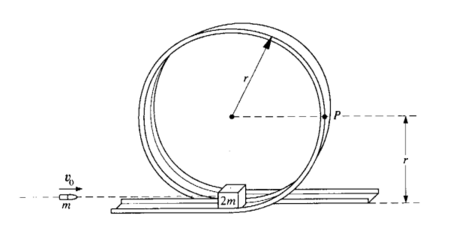# Problem: A small block of mass 2m initially rests on a track at the bottom of the circular, vertical loop-the-loop shown above, which has a radius r. The surface contact between the block and the loop is frictionless. A bullet of mass m strikes the block horizontally with initial speed v0 and remains embedded in the block as the block and bullet circle the loop. Determine each of the following in terms of m, v0, r, and g. (a) The speed of the block and bullet immediately after impact (b) The kinetic energy of the block and bullet when they reach point P on the loop(c) The minimum initial speed vmin of the bullet if the block and bullet are to successfully execute a complete circuit of the loop

###### FREE Expert Solution

We have a collision (conservation of momentum), kinetic energy, and gravitational potential energy.

Conservation of momentum:

$\overline{){{\mathbf{m}}}_{{\mathbf{1}}}{{\mathbf{v}}}_{{\mathbf{01}}}{\mathbf{+}}{{\mathbf{m}}}_{{\mathbf{2}}}{{\mathbf{v}}}_{{\mathbf{02}}}{\mathbf{=}}{{\mathbf{m}}}_{{\mathbf{1}}}{{\mathbf{v}}}_{\mathbf{f}\mathbf{1}}{\mathbf{+}}{{\mathbf{m}}}_{{\mathbf{2}}}{{\mathbf{v}}}_{\mathbf{f}\mathbf{2}}}$

Conservation of energy:

$\overline{){{\mathbf{K}}}_{{\mathbf{0}}}{\mathbf{+}}{{\mathbf{U}}}_{{\mathbf{0}}}{\mathbf{+}}{{\mathbf{W}}}_{{\mathbf{nc}}}{\mathbf{=}}{{\mathbf{K}}}_{{\mathbf{f}}}{\mathbf{+}}{{\mathbf{U}}}_{{\mathbf{f}}}}$, where Wnc is the work done by non-conservative forces such as friction.

(a)

We'll use conservation of momentum.

Let the bullet be 1 and block 2:

m1v01 + m2v02 = m1vf1 + m2vf2

bullet and block fuse to make a single mass;

87% (25 ratings)###### Problem DetailsA small block of mass 2m initially rests on a track at the bottom of the circular, vertical loop-the-loop shown above, which has a radius r. The surface contact between the block and the loop is frictionless. A bullet of mass m strikes the block horizontally with initial speed v0 and remains embedded in the block as the block and bullet circle the loop. Determine each of the following in terms of m, v0, r, and g.

(a) The speed of the block and bullet immediately after impact

(b) The kinetic energy of the block and bullet when they reach point P on the loop

(c) The minimum initial speed vmin of the bullet if the block and bullet are to successfully execute a complete circuit of the loop

Frequently Asked Questions

What scientific concept do you need to know in order to solve this problem?

Our tutors have indicated that to solve this problem you will need to apply the Collisions & Motion (Momentum & Energy) concept. You can view video lessons to learn Collisions & Motion (Momentum & Energy). Or if you need more Collisions & Motion (Momentum & Energy) practice, you can also practice Collisions & Motion (Momentum & Energy) practice problems.